# Summer and Subtractor OpAmp Circuits

• #### Question 1

 Don’t just sit there! Build something!!

Learning to mathematically analyze circuits requires much study and practice. Typically, students practice by working through lots of sample problems and checking their answers against those provided by the textbook or the instructor. While this is good, there is a much better way.

You will learn much more by actually building and analyzing real circuits, letting your test equipment provide the “answers” instead of a book or another person. For successful circuit-building exercises, follow these steps:

1. Carefully measure and record all component values prior to circuit construction.
2. Draw the schematic diagram for the circuit to be analyzed.
3. Carefully build this circuit on a breadboard or other convenient medium.
4. Check the accuracy of the circuit’s construction, following each wire to each connection point, and verifying these elements one-by-one on the diagram.
5. Mathematically analyze the circuit, solving for all voltage and current values.
6. Carefully measure all voltages and currents, to verify the accuracy of your analysis.
7. If there are any substantial errors (greater than a few percent), carefully check your circuit’s construction against the diagram, then carefully re-calculate the values and re-measure.

Avoid using the model 741 op-amp, unless you want to challenge your circuit design skills. There are more versatile op-amp models commonly available for the beginner. I recommend the LM324 for DC and low-frequency AC circuits, and the TL082 for AC projects involving audio or higher frequencies.

As usual, avoid very high and very low resistor values, to avoid measurement errors caused by meter “loading”. I recommend resistor values between 1 kΩ and 100 kΩ.

One way you can save time and reduce the possibility of error is to begin with a very simple circuit and incrementally add components to increase its complexity after each analysis, rather than building a whole new circuit for each practice problem. Another time-saving technique is to re-use the same components in a variety of different circuit configurations. This way, you won’t have to measure any component’s value more than once.

• #### Question 2

The simple resistor network shown here is known as a passive averager. Describe what the word “passive” means in this context, and write an equation describing the output voltage (Vd) in terms of the input voltages (Va, Vb, and Vc):Hint: there is a network theorem that directly applies to this form of circuit, and it is known as Millman’s Theorem. Research this theorem and use it to generate your equation!

• #### Question 3

Add an op-amp circuit to the output of this passive averager network to produce a summer circuit: an operational circuit generating an output voltage equal to the sum of the four input voltages. Then, write an equation describing the whole circuit’s function.• #### Question 4

Write a mathematical equation for this op-amp circuit, assuming all resistor values are equal:What is this circuit typically called?

• #### Question 5

This opamp circuit is known as a difference amplifier, sometimes called a subtractor. Assuming that all resistor values are equal in the circuit, write an equation expressing the output (y) as a function of the two input voltages (a and b):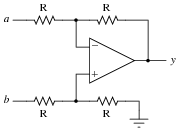• #### Question 6

Determine all current magnitudes and directions, as well as voltage drops, in this circuit:• #### Question 7

Determine all current magnitudes and directions, as well as voltage drops, in this circuit:• #### Question 8

Determine the amount of current from point A to point B in this circuit: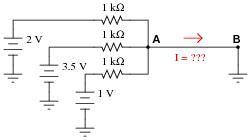• #### Question 9

Determine the amount of current from point A to point B in this circuit, and also the output voltage of the operational amplifier: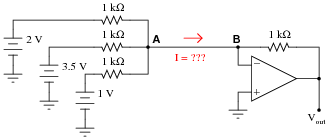• #### Question 10

Identify some of the distinguishing characteristics of inverting and non-inverting summer circuits. How may you identify which is which, and how may you determine the proper resistor values to make each one work as it should?

• #### Question 11

Complete the table of values for this opamp circuit, calculating the output voltage for each combination of input voltages shown:V1 V2 Vout 0 V 0 V 1 V 0 V 0 V 1 V 2 V 1.5 V 3.4 V 1.2 V -2 V 4 V 5 V 5 V -3 V -3 V

What pattern do you notice in the data? What mathematical relationship is there between the two input voltages and the output voltage?

• #### Question 12

How does the operation of this difference amplifier circuit compare with the resistor values given (2R = twice the resistance of R), versus its operation with all resistor values equal?Describe what approach or technique you used to derive your answer, and also explain how your conclusion for this circuit might be generalized for all difference amplifier circuits.

• #### Question 13

If a weak voltage signal is conveyed from a source to an amplifier, the amplifier may detect more than just the desired signal. Along with the desired signal, external electronic “noise” may be coupled to the transmission wire from AC sources such as power line conductors, radio waves, and other electromagnetic interference sources. Note the two waveshapes, representing voltages along the transmission wire measured with reference to earth ground: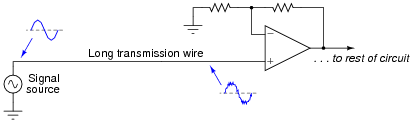Shielding of the transmission wire is always a good idea in electrically noisy environments, but there is a more elegant solution than simply trying to shield interference from getting to the wire. Instead of using a single-ended amplifier to receive the signal, we can transmit the signal along two wires and use a difference amplifier at the receiving end. Note the four waveforms shown, representing voltages at those points measured with reference to earth ground: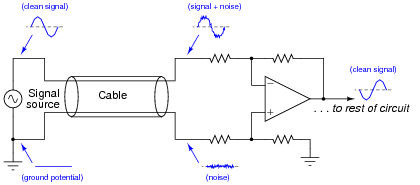If the two wires are run parallel to each other the whole distance, so as to be exposed to the exact same noise sources along that distance, the noise voltage at the end of the bottom wire will be the same noise voltage as that superimposed on the signal at the end of the top wire.

Explain how the difference amplifier is able to restore the original (clean) signal voltage from the two noise-ridden voltages seen at its inputs with respect to ground, and also how the phrase common-mode voltage applies to this scenario.

• #### Question 14

Singers who wish to practice singing to popular music find that the following vocal eliminator circuit is useful: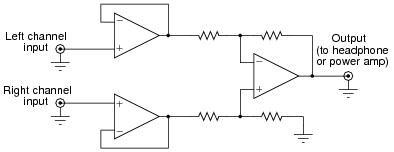The circuit works on the principle that vocal tracks are usually recorded through a single microphone at the recording studio, and thus are represented equally on each channel of a stereo sound system. This circuit effectively eliminates the vocal track from the song, leaving only the music to be heard through the headphone or speaker.

Explain how the operational amplifiers accomplish this task of vocal track elimination. What role does each opamp play in this circuit?

• #### Question 15

The following circuit is known as an instrumentation amplifier:Suppose a DC voltage were to be applied to the non-inverting input terminal, 1 volt at Vin( ), and the inverting input terminal grounded. Complete the following table showing the output voltage of this circuit for different values of m:

 m Vout 1 2 3 4 5 6

• #### Question 16

Find the datasheet for a real instrumentation amplifier (packaged as a single integrated circuit) and bring it to class for discussion with your classmates. Analyze and discuss the inner workings of the circuit, and some of its performance parameters. If you do not know where to begin looking, try researching the Analog Devices model AD623, either in a reference book or on the internet.

• #### Question 17

The following circuit is a type of difference amplifier, similar in behavior to the instrumentation amplifier, but only using two operational amplifiers instead of three: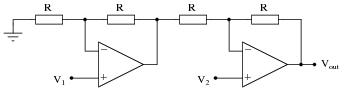Complete the table of values for this opamp circuit, calculating the output voltage for each combination of input voltages shown. From the calculated values of output voltage, determine which input of this circuit is inverting, and which is non-inverting, and also how much differential voltage gain this circuit has. Express these conclusions in the form of an equation.

 V1 V2 Vout 0 V 0 V 1 V 0 V 0 V 1 V 2 V 1.5 V 3.4 V 1.2 V -2 V 4 V 5 V 5 V -3 V -3 V

• #### Question 18

An important parameter of any differential amplifier - bare opamps and difference amplifiers made from opamps alike - is common-mode rejection, or CMR. Explain what this parameter means, how the following circuit tests this parameter, and why it is important to us:• #### Question 19

Explain what common-mode rejection ratio means for a differential amplifier, and give a formula for calculating it.

• #### Question 20

Predict how the operation of this passive averager network will be affected as a result of the following faults. Consider each fault independently (i.e. one at a time, no multiple faults):Resistor R1 fails open:
Solder bridge (short) across resistor R1:
Resistor R2 fails open:
Solder bridge (short) across resistor R2:
Resistor R3 fails open:
Solder bridge (short) across resistor R3:

For each of these conditions, explain why the resulting effects will occur.

• #### Question 21

Predict how the operation of this summer circuit will be affected as a result of the following faults. Consider each fault independently (i.e. one at a time, no multiple faults):Resistor R1 fails open:
Solder bridge (short) across resistor R3:
Resistor R4 fails open:
Resistor R5 fails open:
Solder bridge (short) across resistor R5:
Resistor R6 fails open:

For each of these conditions, explain why the resulting effects will occur.

• #### Question 22

Predict how the operation of this summer circuit will be affected as a result of the following faults. Consider each fault independently (i.e. one at a time, no multiple faults):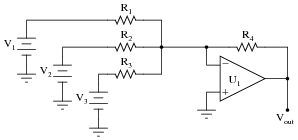Resistor R1 fails open:
Resistor R2 fails open:
Solder bridge (short) across resistor R3:
Resistor R4 fails open:
Solder bridge (short) across resistor R4:

For each of these conditions, explain why the resulting effects will occur.

• #### Question 23

Predict how the operation of this difference amplifier circuit will be affected as a result of the following faults. Consider each fault independently (i.e. one at a time, no multiple faults):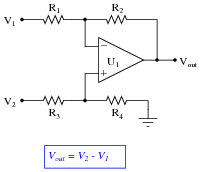Resistor R1 fails open:
Resistor R2 fails open:
Solder bridge (short) across resistor R3:
Resistor R4 fails open:
Solder bridge (short) across resistor R4:

For each of these conditions, explain why the resulting effects will occur.

• #### Question 24

The instrumentation amplifier is a popular circuit configuration for analog signal conditioning in a wide variety of electronic measurement applications. One of the reasons it is so popular is that its differential gain may be set by changing the value of a single resistor, the value of which is represented in this schematic by a multiplier constant named m: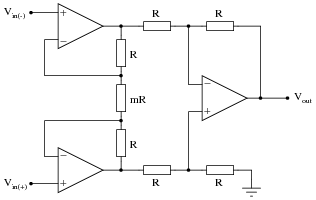There is an equation describing the differential gain of an instrumentation amplifier, but it is easy enough to research so I’ll leave that detail up to you. What I’d like you to do here is algebraically derive that equation based on what you know of inverting and non-inverting operational amplifier circuits.

Suppose we apply 1 volt to the non-inverting input and ground the inverting input, giving a differential input voltage of 1 volt. Whatever voltage appears at the output of the instrumentation amplifier circuit, then, directly represents the voltage gain:A hint for constructing an algebraic explanation for the circuit’s output voltage is to view the two “buffer” opamps separately, as inverting and non-inverting amplifiers:Note which configuration (inverting or non-inverting) each of these circuits resemble, develop transfer functions for each (Output = … Input), then combine the two equations in a manner representing what the subtractor circuit will do. Your final result should be the gain equation for an instrumentation amplifier in terms of m.

• #### Question 25

Calculate the voltage gain of the following opamp circuit with the potentiometer turned fully up, precisely mid-position, and fully down:AV (pot fully up) =
AV (pot mid-position) =
AV (pot fully down) =